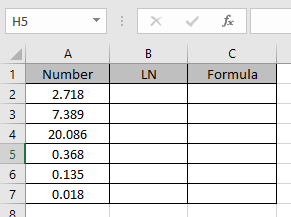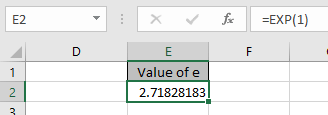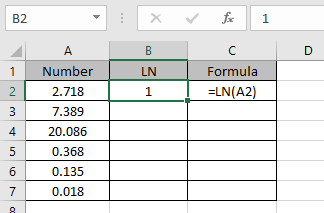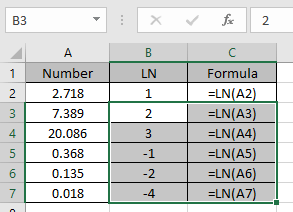# How to use the Excel LN function

In this article, we will learn about how to use the LN function in Excel.

In mathematics ln is an inverse of an exponential function. The logarithm to base e (value of e is approximately equals to 2.7128) is called the natural logarithm.
ex= y
x = loge(y)
The LN function returns the log of a value at base e. For example log(e) at base e returns 1.
Syntax:

=LN(number)

Note: it works the same as LOG function where base is fixed to constant “e”.

Let’s understand this function using it an example.
Here we have numbers in Column A. we need to find the LN of these numbers.These numbers are generated using EXP functions as we can’t get the constant value of “e” in Excel.Use the formula

=LN(A2)

Here LN returns the Logarithmic value in A2 at base “e”.e1= 2.7128..
1 = loge(e)
The above stated equation stats that LN(e) = 1

Now we will apply the formula to other cells to get the LN of all numbers.As you can see LN function returns the logarithmic of the numbers at base e.

Hope you understood how to use LN function and referring cell in Excel. Explore more articles on Excel mathematical function function here. Please feel free to state your query or feedback for the above article.

Related Article:

How to use the LOG function in Excel

How to use the Excel LOG10 function

Popular Articles:

How to use the VLOOKUP Function in Excel

How to use the COUNTIF function in Excel 2016

How to Use SUMIF Function in Excel

Terms and Conditions of use

The applications/code on this site are distributed as is and without warranties or liability. In no event shall the owner of the copyrights, or the authors of the applications/code be liable for any loss of profit, any problems or any damage resulting from the use or evaluation of the applications/code.